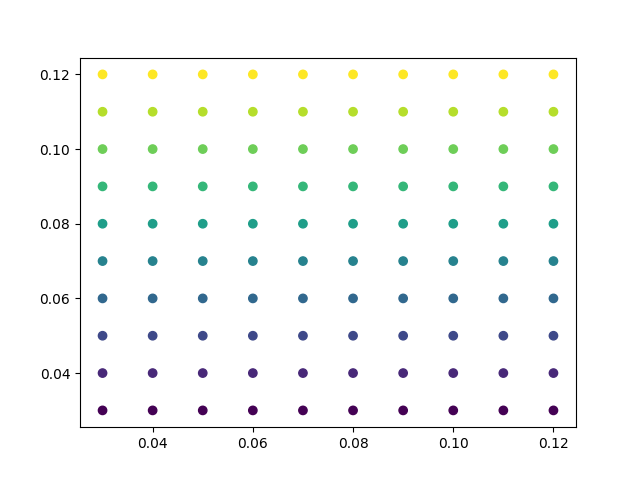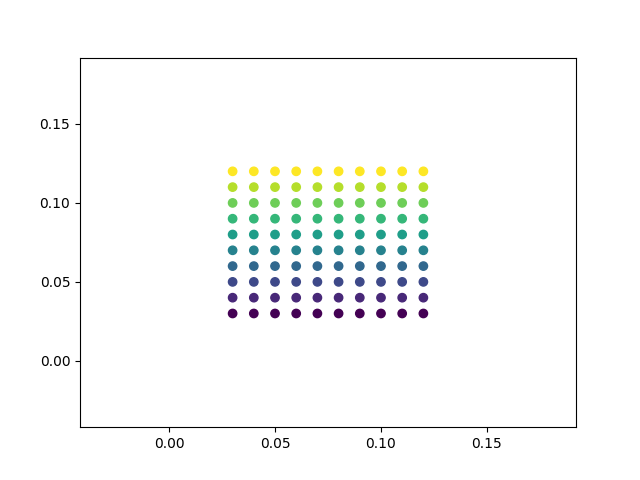# Automatically setting tick labels¶

Setting the behavior of tick auto-placement.

If you don't explicitly set tick positions / labels, Matplotlib will attempt to choose them both automatically based on the displayed data and its limits.

By default, this attempts to choose tick positions that are distributed along the axis:

import matplotlib.pyplot as plt
import numpy as np
np.random.seed(19680801)

fig, ax = plt.subplots()
dots = np.arange(10) / 100. + .03
x, y = np.meshgrid(dots, dots)
data = [x.ravel(), y.ravel()]
ax.scatter(*data, c=data)Out:

<matplotlib.collections.PathCollection object at 0x7f08b719e100>


Sometimes choosing evenly-distributed ticks results in strange tick numbers. If you'd like Matplotlib to keep ticks located at round numbers, you can change this behavior with the following rcParams value:

print(plt.rcParams['axes.autolimit_mode'])

# Now change this value and see the results
with plt.rc_context({'axes.autolimit_mode': 'round_numbers'}):
fig, ax = plt.subplots()
ax.scatter(*data, c=data)Out:

data


You can also alter the margins of the axes around the data by with axes.(x,y)margin:

with plt.rc_context({'axes.autolimit_mode': 'round_numbers',
'axes.xmargin': .8,
'axes.ymargin': .8}):
fig, ax = plt.subplots()
ax.scatter(*data, c=data)

plt.show()Keywords: matplotlib code example, codex, python plot, pyplot Gallery generated by Sphinx-Gallery